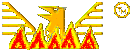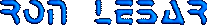©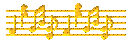Music technology Formulae & equations DeciBels, basic table Small ratio tables Origins & derivations deciBels & volume ratios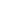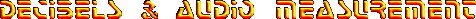DeciBels, power ratios & audio measurement.
For electrical engineering, electronics & music technology.

Voltges ratio columns also apply to current ratios.
Apology. Arbitrary figures accidentally left in the small values table are now corrected.Ratios denoted by the most common deciBel values. dB Voltage Power dB Voltage Power 0.5 1.06 1.12 25 17.8 316.23 1 1.12 1.26 30 31.623 1000 2 1.26 1.58 35 56.29 3,162.3 3 1.414 2.00 40 100 10,000 4 1.58 2.51 50 316.23 105 5 1.78 3.162 60 1000 106 6 1.99 3.98 70 3162.3 107 7 2.24 5.01 80 104 108 8 2.51 6.31 90 31,623 109 9 2.82 7.94 100 105 1010 10 3.162 10 110 316.233 1011 12 3.98 15.8 120 106 1012 15 5.62 31.62 130 316.234 1013 20 10 100 140 107 1014

The deciBel is one of the commonly misundertood aspects of sound & electrical measurement.

Not a unit of measurement as such, but a convenient means of specifying the relationship between 2 values.

The Bel, named after Alexander Graham Bell, is the common logarithm of a power or sound intensity ratio.

Fractional exponents are commonly encountered. To avoid this, the deciBel (dB), 1 tenth of a Bel, is used.

This table covers the most useful range for music & audio purposes.

Given a specified impedance Voltage or current varies as the square root of power. The Voltage ratio columns also apply to current in this case. So 20dB (2 Bels) is a power ratio of 10 to the power of 2 (100). The Voltage & current ratio is the square root, or just 10 (101).

For values not in the table the deciBels can be added & the ratios multiplied. For example, to find a power ratio of 5,000 to 1: 1,000 is 30dB & 5 is roughly 7dB. So the answer is 37dB, within the limitations of this table. Conversely 28dB is 8dB + 20 dB, the Voltage ratio is therefore 2.51 X 10 or 25.1 to 1.

For greater accuracy with small ratios another table will be included at the end of this section. Feel free to download these tables if they are of use. We hope at some point to include the complete set of Henry Briggs logarithm tables.

The table clearly shows, with multiples of 10dB, that taking off the last zero gives the exponent for power ratios. The origin of the system lies in the realm of telephony. Here matching impedances for maximum power transfer is all important. This is why it relates directly to power rather than Voltage or current.

Originally called the Transmission Unit (T.U.), the name was changed in the 1920s. Early valve (vacuum tube) equipment has similiar requirements so the deciBel was adopted quickly throughout the sound engineering field in Britain & America.

Continental Europe had a similiar system called the Neper, from the original spelling of John Napier's (1550 to 1617) surname. He gave the world logarithms in 1614 & his version, to base e, is the basis of the Neper. It is thus a little smaller than the Bel.

It is interesting to note that common logarithms, to base 10 with log 1 = 0, arose out of discussions with Henry Briggs, in 1615 & 1616. Mr. Briggs published his first common log tables in 1617, giving credit to John Napier.

Within a few years a complete set were published, for all natural numbers (positive integers) from 1 to 100,000. Thes tables are to 14 places of decimals, better than most calculators can manage today. John Napier was also the man who gave us decimal notation.

Decimals were later adopted in America, for their currency after independence & by France after their revolution. Despite the system's advantages it only took over here in the second half of the 20th Century. The advent of cheap calculators finally ousted fractions from the academic mainstream. Some early 'mathematical' calculators had fractional input ability.Audio measurement using deciBels

When the second term of the ratio is replaced by a constant, the deciBel becomes a unit of measurement. A number of such systems are in common use.

dBm, a measurement system relative to 1 milliWatt into 600Ω, the m stands for 'milliWatt'. The Voltage corresponding to this is 0.7746 Volts RMS. Stage instruments & instruments usually work at -10dBm. Studios & broadcasting use +4dBm as a normal level.

dBu, a similiar standard where 0dBu is 0.7746 Volts into any impedance, the u stands for 'unterminated'. Useful with more modern transistor equipment where impedance is less important.

dBv, an earlier name for dBu, the small v stands for Volts. No longer used as many writers etc. confused it with dBV (capital V).

dBV, similiar to dBu, but the standard reference is one Volt, the capital V stands for Volt. Our Bruel & Kjaer test equipment uses this standard.

VU, Volume Unit, a system for use with averaging sound level meters. Calibration is similiar to dBm, but the correlation only holds for steady sine waves. Normally set so that 0VU is +4dBm. These meters are of little use with digital recording.

dBW, a power measurement system where the reference standard is 1 Watt. Used for higher power systems & is less common.

dBK, in this standard 0dBK is one KiloWatt (1000 Watts). It is hard to see where this fits into most music use.

dBFS, a standard for digital recording, FS stands for Full Scale. 0dBFS is the level at which all bits of the digital code are set to 1, The signal can not get any greater than this, digital systems have zero headroom. For reproduction without clipping, the peak signal must be less than or equal to 0dBFS. Thus all working levels are negative figures.dBi, my proposed name for the unit of sound intensity, the i stands for intensity. The dB name is often quoted for 'unweighted' measurement. As this is the established name for a dimensionless ratio, it is confusion by & for the uneducated. A 20dB increase can change from loud to deafening, a 20dB level is very quiet. Where is the sense in that?

0dBi (or 0dB), the reference level, is 1 picoWatt (1 Watt x 10-12) per square metre. This miniscule figure is the measured threshold of hearing for a healthy young human @ 1 Kc/s. This is the acoustic equivalent of electrical power.

The level at which hearing damage can occur is 120dBi, one acoustic Watt. This is 1012, 1 Imperial billion (American trillion) times greater. So the range of human hearing is quite wide, I don't know how many people volunteered to go deaf to get this figure. One of the reasons for using a logarithmic scale is this wide range of hearing perception.

dBA, a similiar measure, but with the reference set to a curve approximating the human ear's frequency response. two other curves, dBB & dBC are rarely used.

dBSPL, sound pressure level. 0dBSPL is set at 20 microPascals (2 X 10-5 Newtons per square metre), the threshold of hearing. This measure is the equivalent of electrical Volts. 120dBSPL is again the level of potential hearing damage.

Acoustic displacement, or air movement is the equivalent of electrical current. Difficult to measure directly at the frequencies & amplitudes involved. It is approximately calculated from dBSPL readings at two close points, allowing for the specific mass & density of air.

A means of directly measuring minute air displacements at audio frequencies has now been devised. The technique is potentially highly accurate, allthough difficult to calibrate, since no precise standard exists. We may have the nearest thing to a perfect microphone, with essentially similiar mass to air.Smaller deciBel values & relevant ratios. dB Voltage Power dB Voltage Power dB Voltage Power 0.1 1.0116 1.0233 2.0 1.2589 1.5849 0.2 1.0233 1.0471 2.2 1.2882 1.6595 0.3 1.0315 1.0715 2.4 1.3183 1.7328 0.4 1.0471 1.0965 2.6 1.3490 1.8198 0.5 1.0593 1.1220 2.8 1.3804 1.9055 0.6 1.0715 1.1482 3.0 1.4125 1.9953 0.7 1.0839 1.1749 3.5 1.4962 2.2387 0.8 1.0965 1.2023 4.0 1.5849 2.5119 0.9 1.1092 1.2303 4.5 1.6788 2.8184 1.0 1.1220 1.2589 5.0 1.7783 3.1623 1.2 1.1482 1.3183 5.5 1.8836 3.5480 1.4 1.1749 1.3804 6.0 1.9953 3.9811 1.6 1.2023 1.4454 7.0 2.2387 5.0119 1.8 1.2303 1.5136 8.0 2.5119 6.3096Wrong Usage & Confusion.

There is an active campaign to get rid of the good old deciBel that has served us well. It would be replaced by measurements from the SI system of units. The reason is the ignorance & confusion that has plagued the dB for many years. It will be a shame if such a useful system for engineers becomes obsolete.

Just because those who don't know what they are talking about, insist on talking about it. The 'dumbing down' of much of the world's population has found its way into the technical, or pseudo technical writing 'profession'.

As an example take the specification of a typical stage performance loudspeaker driver. The 'sensitivity' figure is of interest, for an efficient model this may be 100dB, by this the writer usually means 100dBA. In some cases the reference power is included, less often the measurement distance, the frequency is seldom mentioned.

If the typical power of 1 Watt is included, it can be inferred that at 100 Watts the threshold of hearing damage (120dBA), will be reached. Exceeded by 3dB at 200 Watts. This is a common misconception, the sensitivity specification is an 'output', not a listener's auditory 'input'. If the distance is given, it is typically 1 Metre for a driver of this power.

No-one will be listening to a 100 or 200 Watt amplifier flat out at 1 metre. In an open space, sound follows the inverse square law, at 2 metres the intensity is one quarter, 6dB dowm. At 10 metres it is one hundredth, 20dB down. In a room full of people &/or soft furnishings sound will be further attenuated. It is reinforced by significant reverberation.We Wish ALL the World Peace, Justice, Equality, Prosperity & an End to Fanaticism.